# DAV Class 5 Maths Chapter 4 Worksheet 3 Solutions

The DAV Class 5 Maths Book Solutions Pdf and DAV Class 5 Maths Chapter 4 Worksheet 3 Solutions of Fractional Numbers offer comprehensive answers to textbook questions.

## DAV Class 5 Maths Ch 4 Worksheet 3 Solutions

Question 1.
Arrange the following in ascending order.
(a) $$\frac{5}{2}, \frac{1}{6}, \frac{3}{4}, \frac{3}{8}$$
Solution: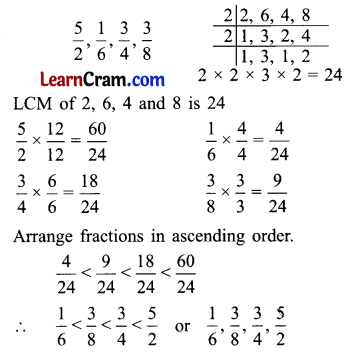(b) $$\frac{2}{9}, \frac{5}{12}, \frac{7}{4}, \frac{1}{6}$$
Solution: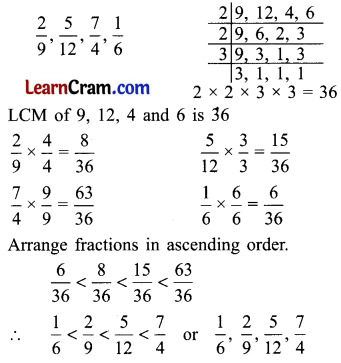(c) $$\frac{11}{15}, \frac{9}{5}, \frac{13}{10}, \frac{7}{20}$$
Solution: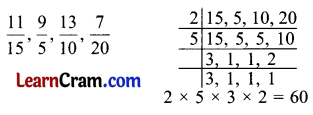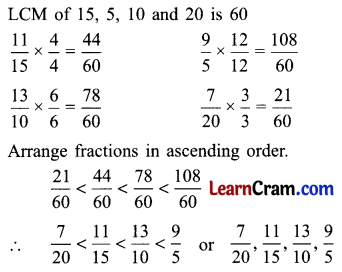(d) $$1 \frac{1}{2}, \frac{11}{6}, 3 \frac{2}{5}, \frac{7}{3}$$
Solution:Question 2.
Arrange the following in descending order.
(a) $$\frac{3}{10}, \frac{7}{15}, \frac{5}{6}, \frac{1}{30}$$
Solution:(b) $$\frac{3}{14}, \frac{7}{5}, \frac{9}{7}, \frac{11}{10}$$
Solution:(c) $$\frac{9}{20}, \frac{2}{10}, \frac{7}{50}, \frac{1}{100}$$
Solution: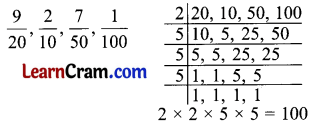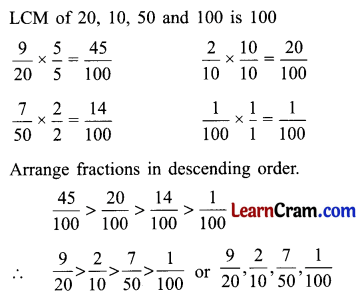(d) $$2 \frac{5}{6}, \frac{7}{18}, 4 \frac{1}{4}, \frac{10}{9}$$
Solution:DAV Class 5 Maths Chapter 4 Worksheet 3 Notes

Arrange, unlike fractions in ascending order.
$$\frac{1}{5}, \frac{5}{3}, \frac{2}{7}, \frac{5}{10}$$
Convert these unlike fractions into like fractions by taking the LCM of the denominators.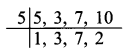5 × 3 × 7 × 2 = 210
LCM of 5, 3, 7 and 10 is
$$\frac{1}{5} \times \frac{42}{42}=\frac{42}{210}$$ (Divide 210 by 5 to get 42)
$$\frac{5}{3} \times \frac{70}{70}=\frac{350}{210}$$ (Divide 210 by 3 to get 70)
$$\frac{2}{7} \times \frac{30}{30}=\frac{60}{210}$$ (Divide 210 by 7 to get 30)
$$\frac{5}{10} \times \frac{21}{21}=\frac{105}{210}$$ (Divide 210 by 10 to get 21)
We arrange these fractions in ascending order.
$$\frac{42}{210}<\frac{60}{210}<\frac{105}{210}<\frac{350}{210}$$ which means, $$\frac{1}{5}<\frac{2}{7}<\frac{5}{10}<\frac{5}{3}$$ or $$\frac{1}{5}, \frac{2}{7}, \frac{5}{10}, \frac{5}{3}$$